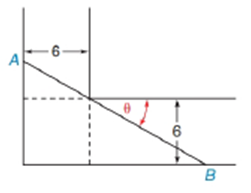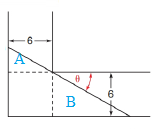Chapter 11.2, Problem 40E### Elementary Geometry for College St...

6th Edition
Daniel C. Alexander + 1 other
ISBN: 9781285195698

#### Solutions

Chapter
Section### Elementary Geometry for College St...

6th Edition
Daniel C. Alexander + 1 other
ISBN: 9781285195698
Textbook Problem
11 views

# A ladder of length AB is carried horizontally through an L-shaped turn in a hallway. Show that the ladder has the length L = 6 sin θ + 6 cos θTo determine

To find:

To show that the ladder has the length L=6sinθ+6cosθ by using the following figure,Explanation

Consider the following figure,

General formula for cosine ratio and sin ratio is given below,

For smaller triangle A:

From the above figure shows that the hypotenuse A of the smaller triangle is formed by the left end of the ladder since it is opposite to right angle

### Still sussing out bartleby?

Check out a sample textbook solution.

See a sample solution

#### The Solution to Your Study Problems

Bartleby provides explanations to thousands of textbook problems written by our experts, many with advanced degrees!

Get Started

#### 2. Find the product. and

Mathematical Applications for the Management, Life, and Social Sciences

#### True or False: x2 + 6x + 2y = 1 defines y as a function of x.

Study Guide for Stewart's Single Variable Calculus: Early Transcendentals, 8th

#### What is the goal of a single-case experimental research design?

Research Methods for the Behavioral Sciences (MindTap Course List)